Examples

Chapter 1 Class 7 Integers
Serial order wise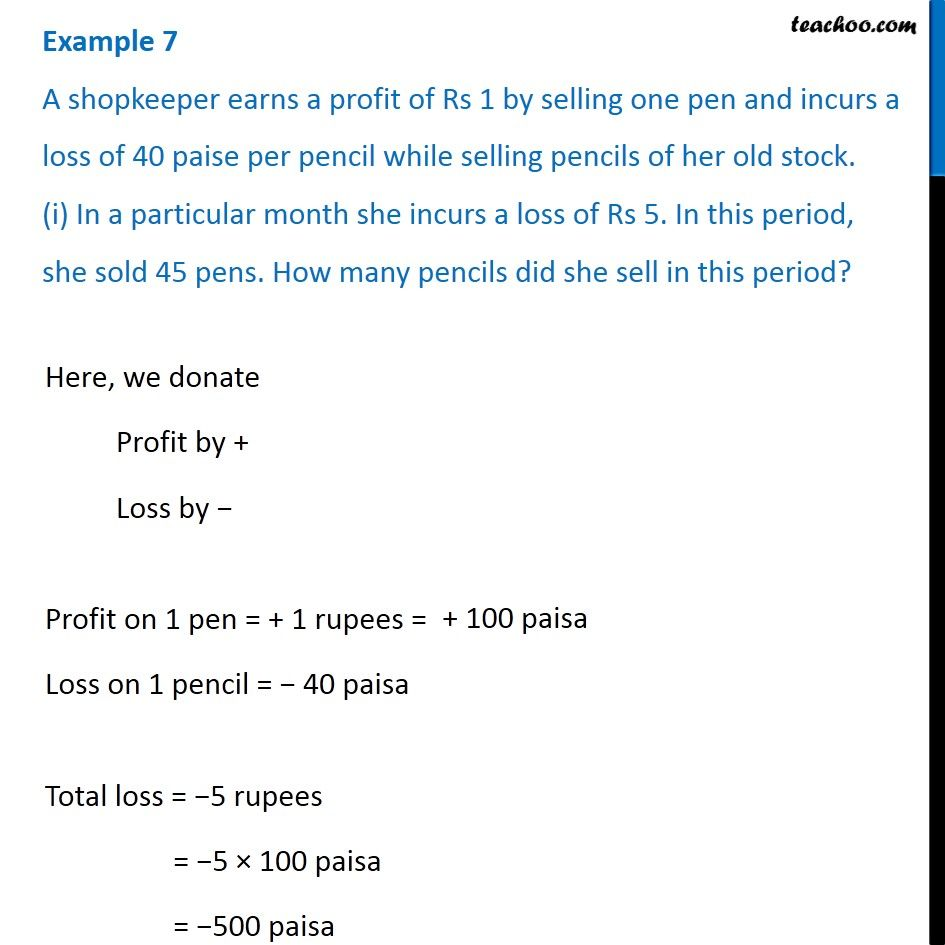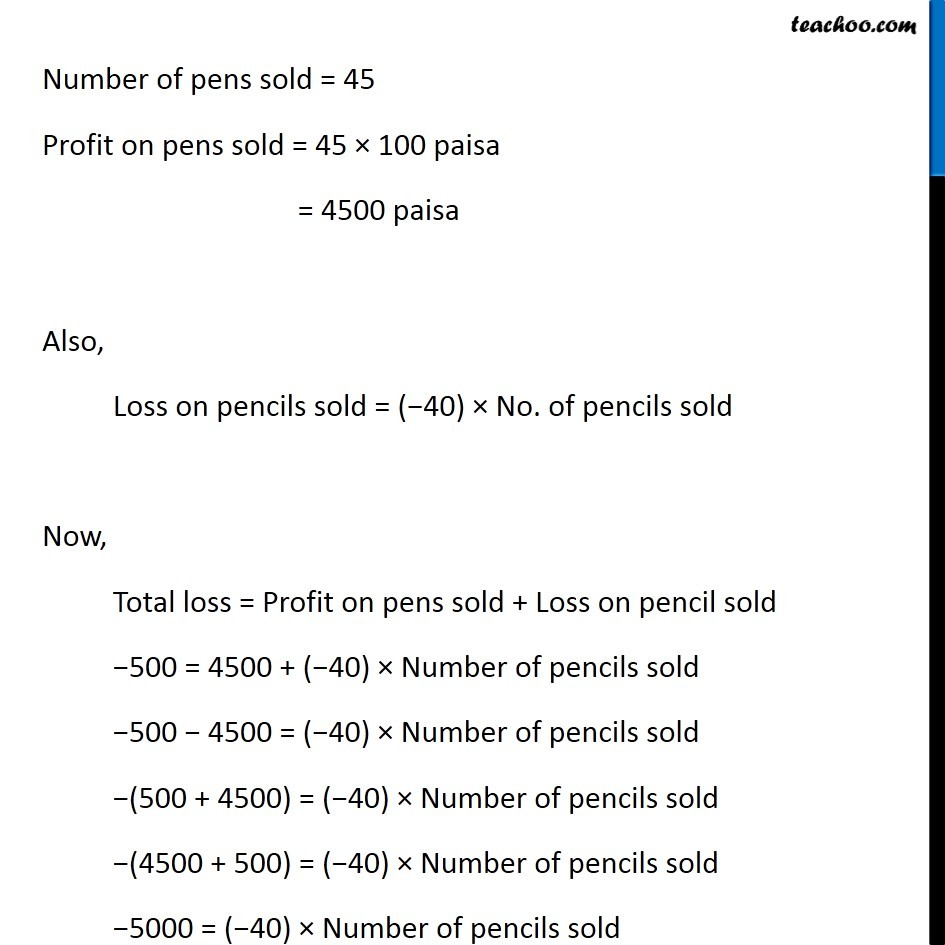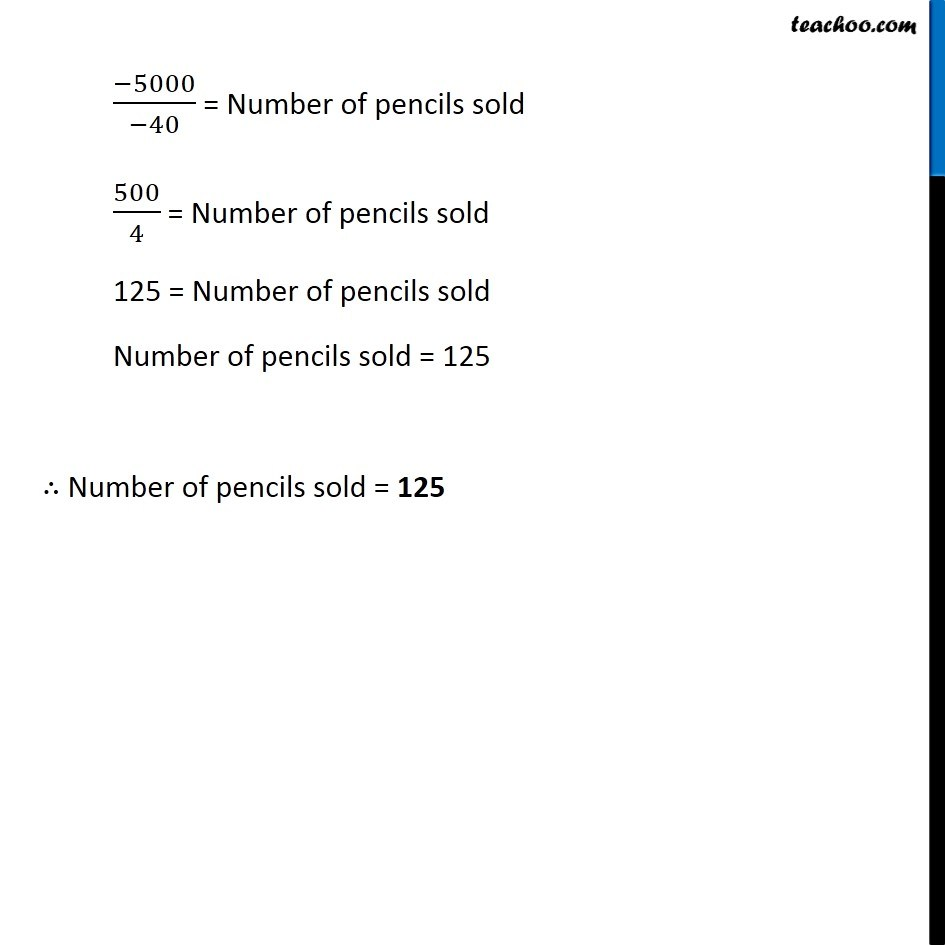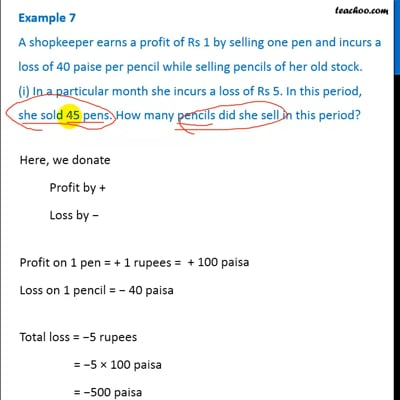This video is only available for Teachoo black users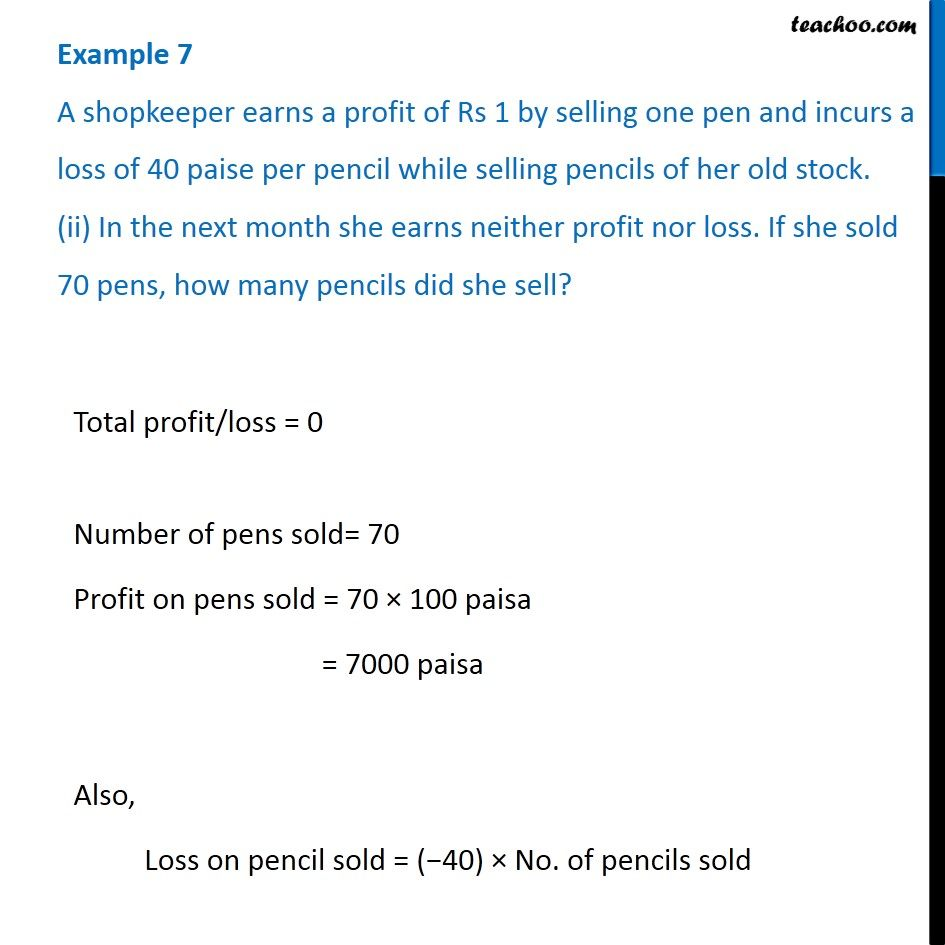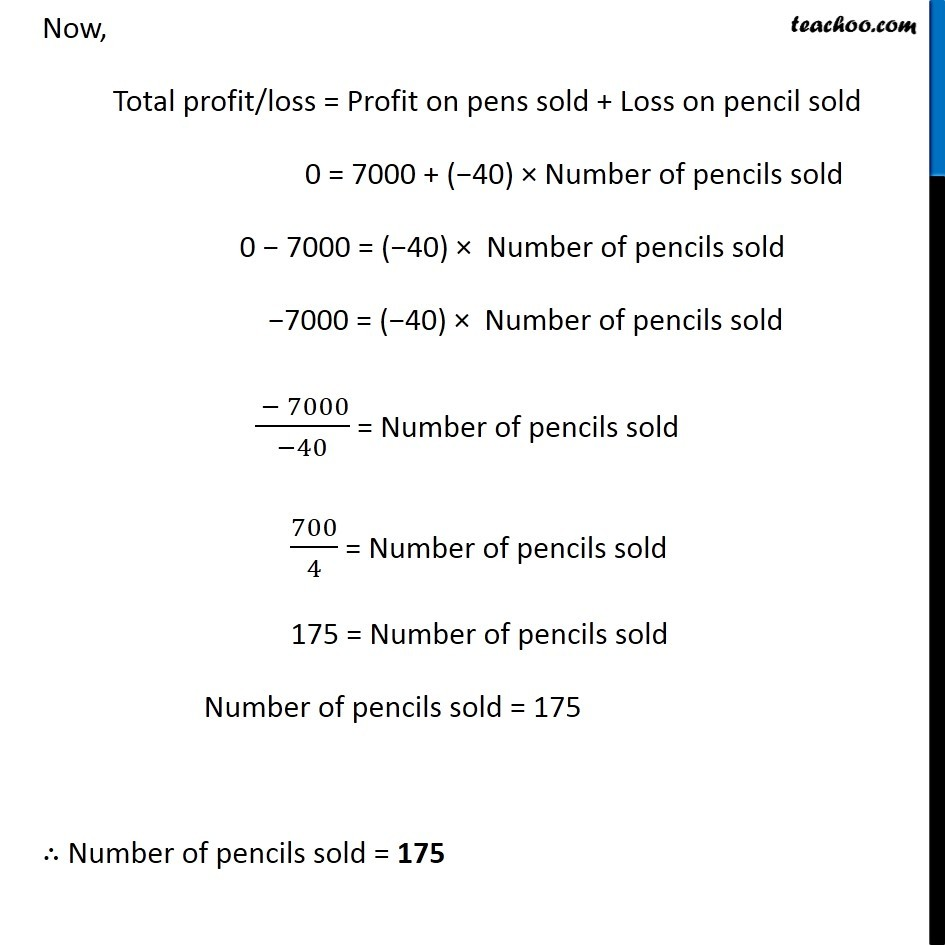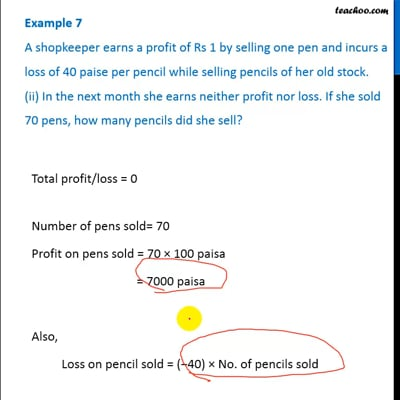This video is only available for Teachoo black users

Get live Maths 1-on-1 Classs - Class 6 to 12

### Transcript

Example 7 A shopkeeper earns a profit of Rs 1 by selling one pen and incurs a loss of 40 paise per pencil while selling pencils of her old stock. (i) In a particular month she incurs a loss of Rs 5. In this period, she sold 45 pens. How many pencils did she sell in this period?Here, we donate Profit by + Loss by − Profit on 1 pen = + 1 rupees = Loss on 1 pencil = − 40 paisa Total loss = −5 rupees = −5 × 100 paisa = −500 paisa Number of pens sold = 45 Profit on pens sold = 45 × 100 paisa = 4500 paisa Also, Loss on pencils sold = (−40) × No. of pencils sold Now, Total loss = Profit on pens sold + Loss on pencil sold −500 = 4500 + (−40) × Number of pencils sold −500 − 4500 = (−40) × Number of pencils sold −(500 + 4500) = (−40) × Number of pencils sold −(4500 + 500) = (−40) × Number of pencils sold −5000 = (−40) × Number of pencils sold (−5000)/(−40) = Number of pencils sold 500/4 = Number of pencils sold 125 = Number of pencils sold Number of pencils sold = 125 ∴ Number of pencils sold = 125 Example 7 A shopkeeper earns a profit of Rs 1 by selling one pen and incurs a loss of 40 paise per pencil while selling pencils of her old stock. (ii) In the next month she earns neither profit nor loss. If she sold 70 pens, how many pencils did she sell?Total profit/loss = 0 Number of pens sold= 70 Profit on pens sold = 70 × 100 paisa = 7000 paisa Also, Loss on pencil sold = (−40) × No. of pencils sold Now, Total profit/loss = Profit on pens sold + Loss on pencil sold 0 = 7000 + (−40) × Number of pencils sold 0 − 7000 = (−40) × Number of pencils sold −7000 = (−40) × Number of pencils sold ( − 7000)/(−40) = Number of pencils sold 700/4 = Number of pencils sold 175 = Number of pencils sold Number of pencils sold = 175 ∴ Number of pencils sold = 175